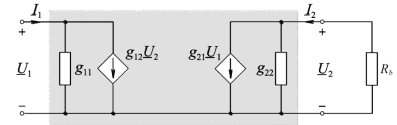# Compute the voltage gain of this bipolar junction transistor circuit

Homework Statement:
Hi!

This is one of the tasks that appeared in our exam preparation script. There is a sample solution path given, but I am unable to understand how to get there.

Idea is that given a bipolar junction transistor (BJT), you need to compute voltage gain ##A_u## from the following parameter values: ##g_{11} = 2mS, g_{12} \approx 0S, g_{21} = 25mS, g_{22} = 0.1mS## and the load has resistence ##R_b = 2500\Omega##.
Relevant Equations:
To solve this task, you only need to remember that voltage gain is computed as $$A_u = \frac{u_2}{u_1}$$ where ##u_2## is the output and ##u_1## is the input voltage.This is the given schematic. The result should be $$A_u = \frac{R_b g_{21}}{1 + R_b g_{22}} = -50$$ and the way I wanted to tackle this problem is by stating $$A_u = \frac{-R_b i_2}{u_1} = \frac{-R_b (g_{21} u_1 + g_{22} u_2)}{u_1} = \frac{-R_b (g_{21} u_1 + g_{22} u_2)}{g_{11} u_1 + g_{12} u_2} = \frac{-R_b g_{21} + g_{22} u_2}{g_{11}}$$ but that leaves me with ##u_2##. I am kind of stuck here.

I do not expect anyone to solve this task for me, I am just looking for guidance on how to continue, what have I missed... Any sort of help will be greatly appreciated!